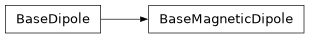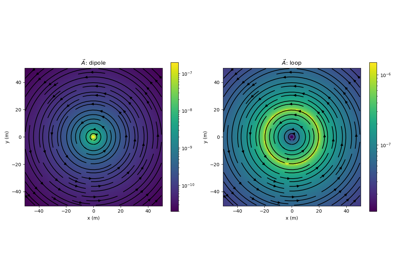# geoana.em.BaseMagneticDipole#class geoana.em.BaseMagneticDipole(moment=1.0, **kwargs)#

Base class for magnetic dipoles.

The BaseMagneticDipole class defines the basic properties for magnetic dipoles. Where $$d\mathbf{m}$$ defines the dipole moment, the magnetic dipole is defined as:

$\mathbf{s_m}(\mathbf{r}) = \mathbf{m} \, \delta(|\mathbf{r} - \mathbf{r_s}|)$

where $$\mathbf{r_s}$$ is the location of the source. For more, visit EM GeoSci .

Parameters
momentfloat, optional

Amplitude of the dipole moment for the magnetic dipole ($$A/m^2$$). Default is 1 $$A/m^2$$.

Attributes

 location Location of the dipole moment Amplitude of the dipole moment of the magnetic dipole ($$A/m^2$$) orientation Orientation of the dipole as a normalized vector

Methods

 Cross products between a gridded set of vectors and the orientation of the source. distance(xyz) Scalar distance from dipole to a set of gridded xyz locations Dot product between the orientation of the source and a gridded set of vectors. Vector distance to a set of gridded xyz locations.

## Galleries and Tutorials using geoana.em.BaseMagneticDipole#Magnetostatic Vector Potentials: Dipole and Loop Sources

Magnetostatic Vector Potentials: Dipole and Loop SourcesTotal magnetic fields: Dipole and Pole sources

Total magnetic fields: Dipole and Pole sources